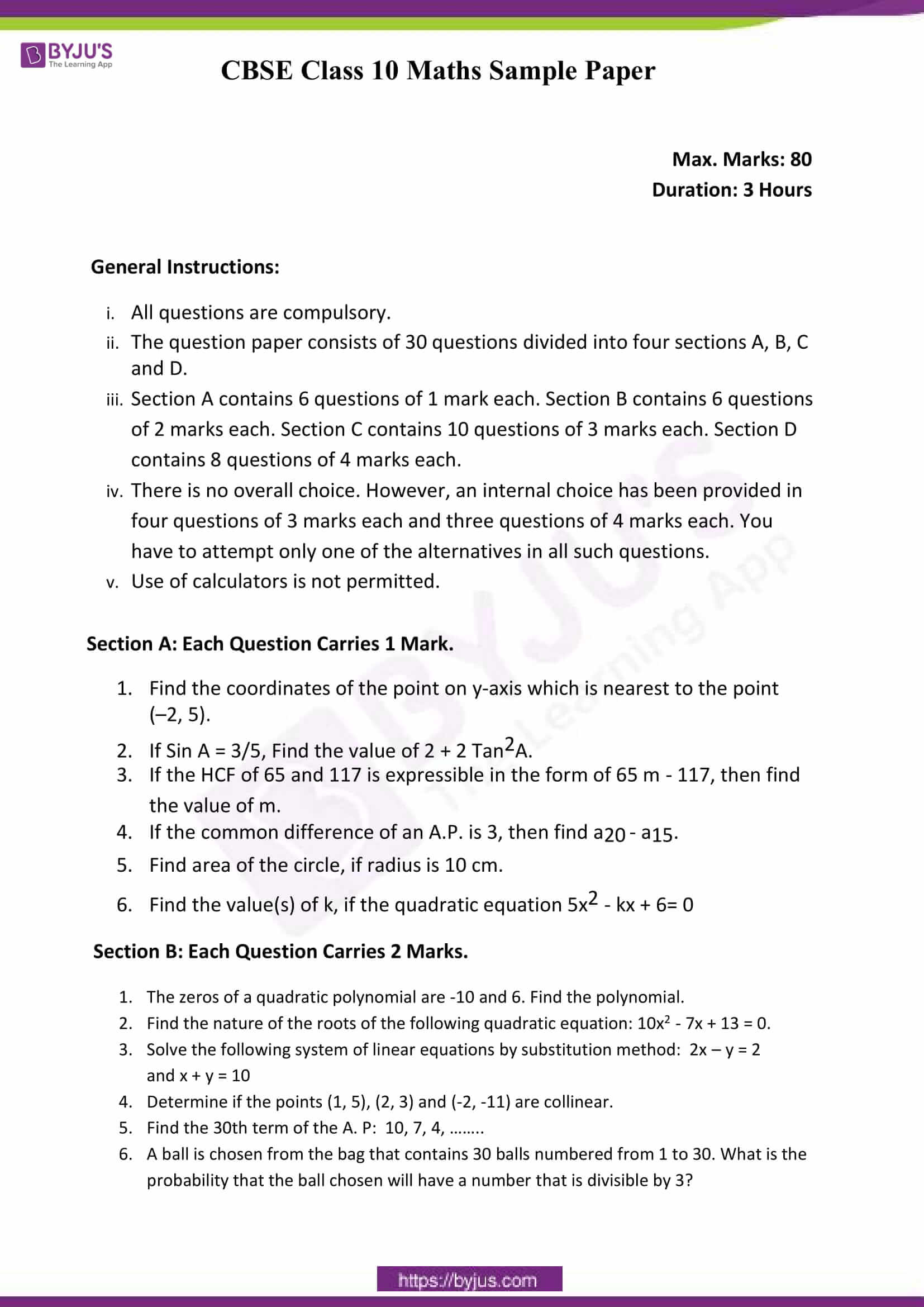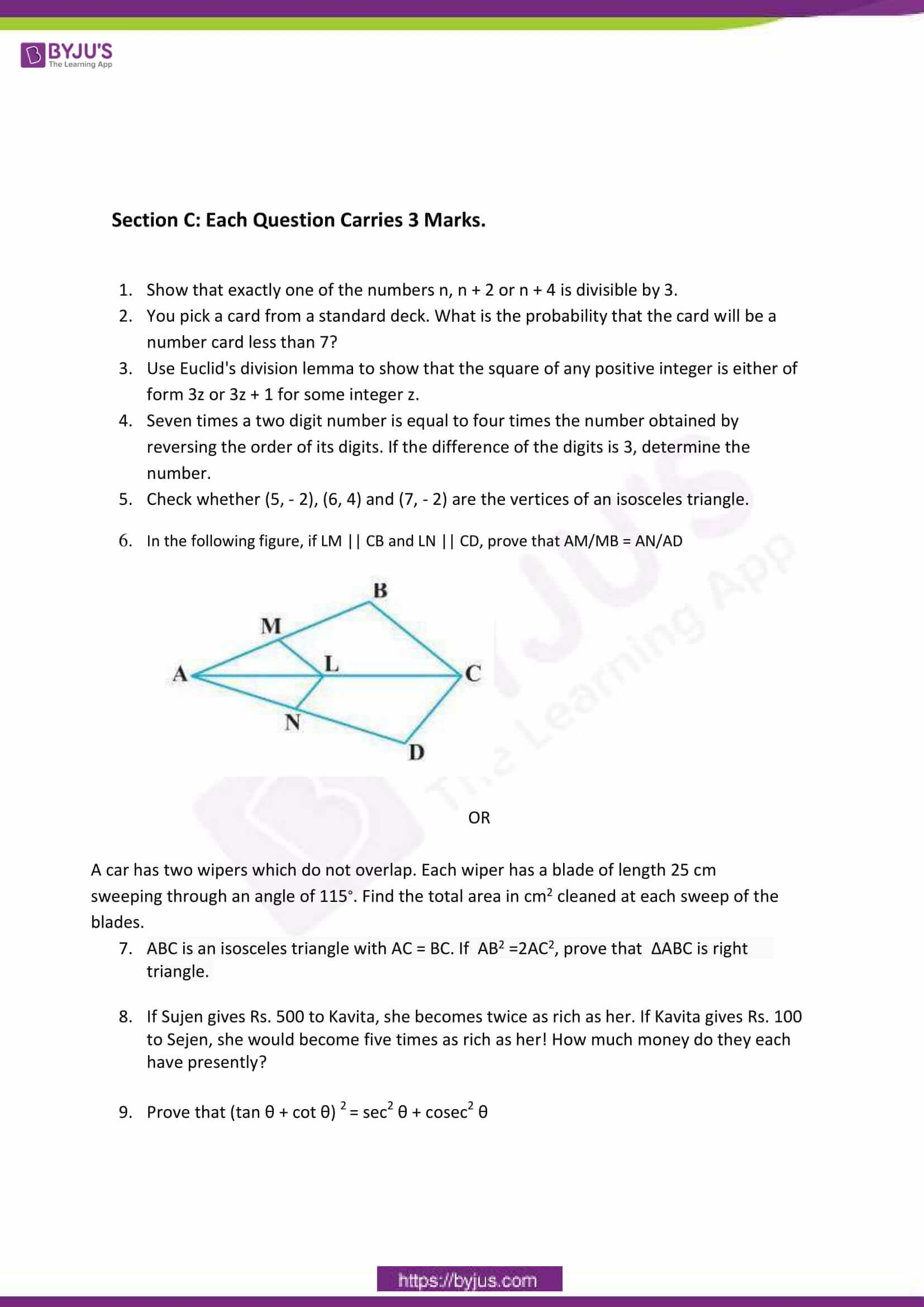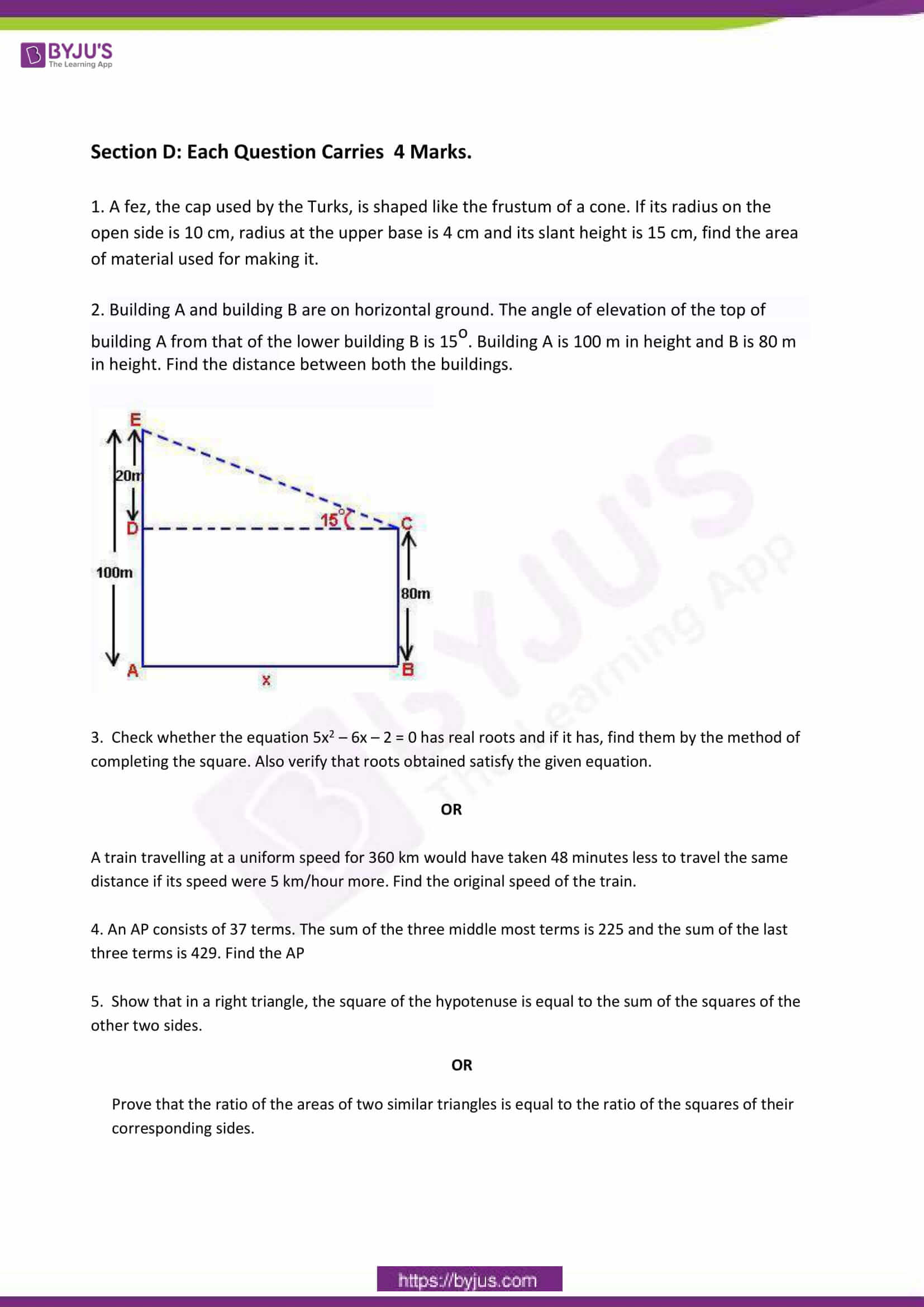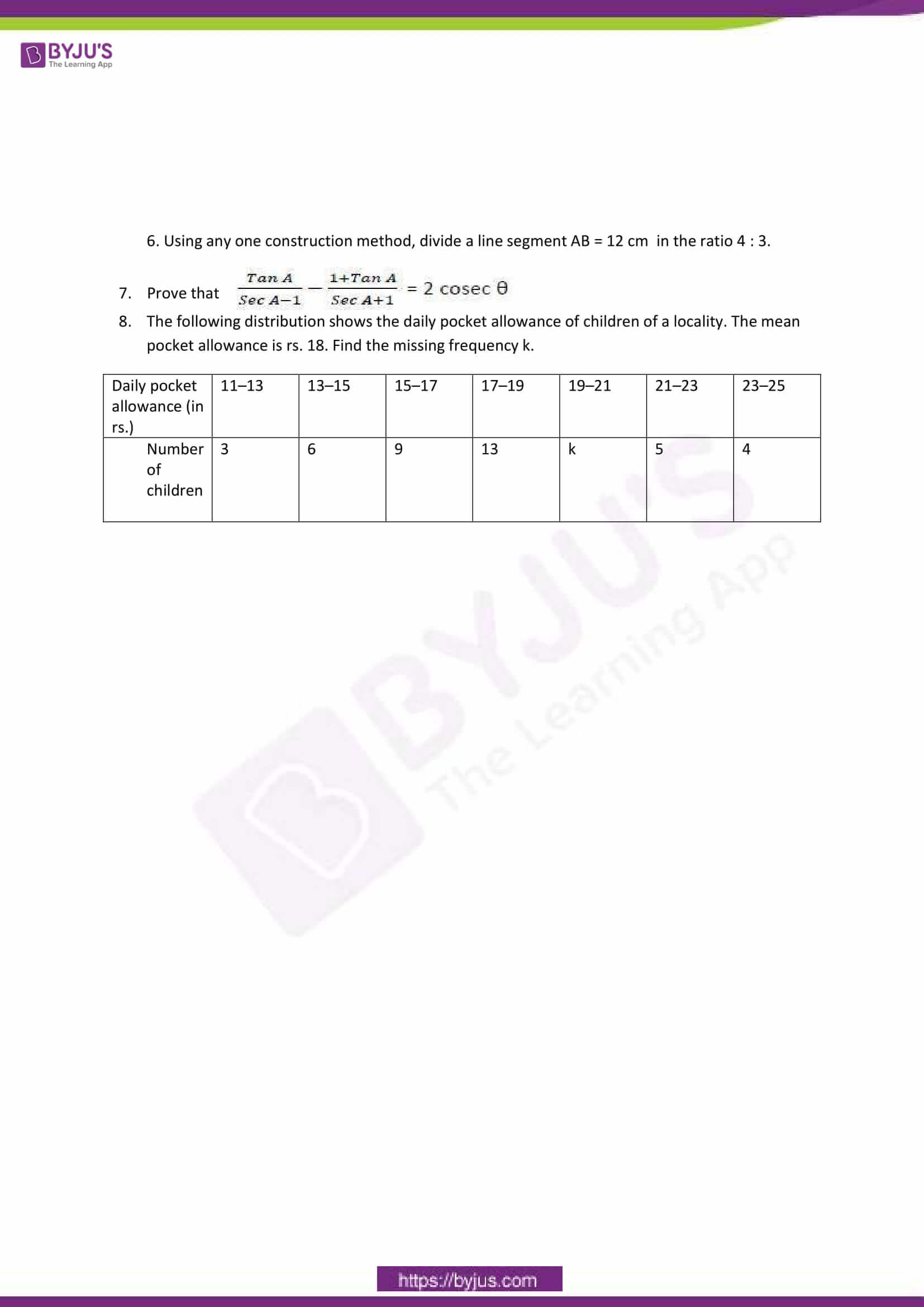# CBSE Class 10 Maths Sample Papers Set 6

One of the best ways to prepare for the Maths exam is to solve sample papers. It helps in understanding the important topics and frequently asked questions from the exam perspective. So, to help in students exam preparation we have provided the CBSE Class 10 Maths Sample Paper Set 6. Solving the CBSE sample paper will enhance the speed, accuracy and time management skills of students. It also gives a proper idea about the examination pattern as well as its difficulty level.### Access Other Sets of CBSE Class 10 Maths Sample Papers

Students can access the other sets of CBSE Class 10 Sample Papers for Maths from the table below:

By practising the CBSE Class 10 Maths Sample Papers, students will get to know how much they are actually prepared to face the board exam. Thus, it will help in evaluating their current preparation level.

### Benefits of Solving the CBSE Class 10 Maths Sample Papers

1. Students get well versed with the exam pattern and marking scheme of the exam.
2. The speed of solving the Maths problem will increase which will help them in finishing the paper on time.
3. It helps in understanding the important topics from where questions are expected to be asked in the exam.
4. Students can analyse their current level of exam preparation.
5. Solving sample papers will boost students confidence.

Keep learning and stay tuned for further updates on CBSE and other competitive exams. Download BYJU’S App and subscribe to YouTube channel to access interactive Maths and Science videos.

 NCERT Related Articles NCERT Solutions for Class 12 NCERT Solutions for Class 11 NCERT Solutions for Class 10 NCERT Solutions for Class 12 Maths NCERT Solutions for Class 11 Maths NCERT Solutions for Class 10 Maths NCERT Solutions for Class 12 Physics NCERT Solutions for Class 11 Physics NCERT Solutions for Class 10 Science NCERT Solutions for Class 12 Chemistry NCERT Solutions for Class 11 Chemistry NCERT Solutions for Class 6 NCERT Solutions for Class 12 Biology NCERT Solutions for Class 11 Biology NCERT Solutions for Class 6 Maths NCERT Solutions for Class 9 NCERT Solutions for Class 8 NCERT Solutions for Class 6 Science NCERT Solutions for Class 9 Maths NCERT Solutions for Class 8 Maths NCERT Solutions for Class 4 NCERT Solutions for Class 9 Science NCERT Solutions for Class 8 Science NCERT Solutions for Class 4 Maths NCERT Solutions for Class 5 NCERT Solutions for Class 7 NCERT Solutions for Class 7 Maths NCERT Solutions for Class 4 Science NCERT Solutions for Class 5 Maths NCERT Solutions for Class 7 Science NCERT Solutions NCERT Books NCERT Solutions for Class 5 Science